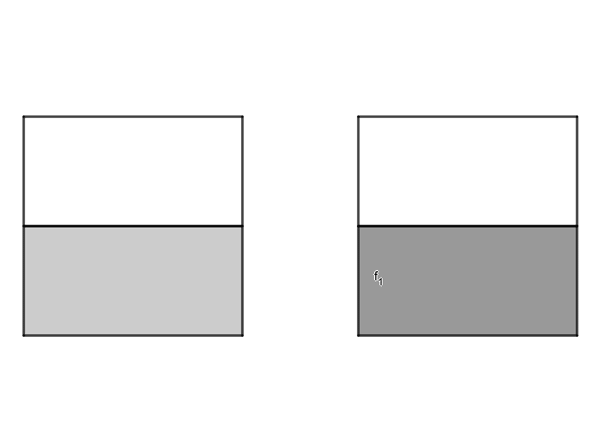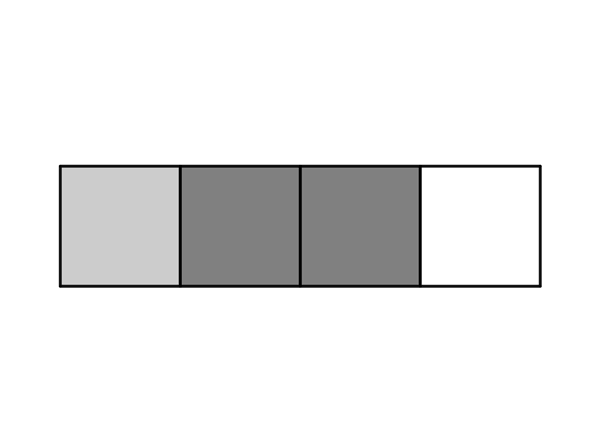Math Calculators, Lessons and Formulas

It is time to solve your math problem

mathportal.org
• Pre algebra
• Fractions

ans:
syntax error
C
DEL
ANS
±
(
)
÷
×
7
8
9
4
5
6
+
1
2
3
=
0
.
auto next question
calculator
•  Question 1: 1 pts Evaluate expression. $$1 + \frac{5}{9}$$
•  Question 2: 1 pts Evaluate each expression. $$\frac{1}{8} +\frac{1}{8} +\frac{5}{8}$$
 $\frac{8}{7}$ $\frac{7}{24}$ $\frac{7}{8}$ $\frac{5}{8}$
•  Question 3: 1 pts Evaluate each expression. $$\frac{12}{15} -\frac{7}{15}$$
 $\frac{5}{0}$ $\frac{8}{7}$ $\frac{5}{15}$ $\frac{1}{5}$
•  Question 4: 1 pts Evaluate each expression. $$\frac{19}{10} -\frac{17}{10}$$
•  Question 5: 2 pts Add the fractions that correspond to the shaded regions. Express the result both as an improper fraction and as a mixed number.$\frac{3}{2}=1\frac{1}{2}$ $\frac{3}{3}=1$ $\frac{2}{2}=1$ none of these
•  Question 6: 2 pts Subtract the mixed numbers. $$2\frac{2}{3}-1\frac{1}{3}$$
•  Question 7: 2 pts Subtract the numbers. $$2- \frac{11}{19}$$
 $1\frac{1}{8}$ $1\frac{8}{19}$ $1\frac{19}{8}$ $1\frac{7}{19}$
•  Question 8: 2 pts Which expression can be used to represent the white regions shown on the picture.$\frac{4}{4} - \frac{3}{4}$ $\frac{4}{4} - \frac{1}{4}$ $\frac{3}{4} - \frac{1}{4}$
•  Question 9: 3 pts Add the fractions $\frac{1}{10} + \frac{2}{15}$
•  Question 10: 3 pts Substract the fractions $3\frac{2}{3} - 2\frac{3}{5}$
 $\frac{16}{15}$ $\frac{15}{16}$ $\frac{14}{15}$ $\frac{15}{14}$
•  Question 11: 3 pts Write the answer in simplest form. $$- \frac{2}{7} + \frac{1}{3}$$
•  Question 12: 3 pts Write the answer in simplest form. $$\frac{9}{5} - \frac{7}{4}+ \frac{4}{4}$$
 $1\frac{6}{5}$ $\frac{1}{9}$ $1\frac{1}{20}$ $\frac{1}{20}$